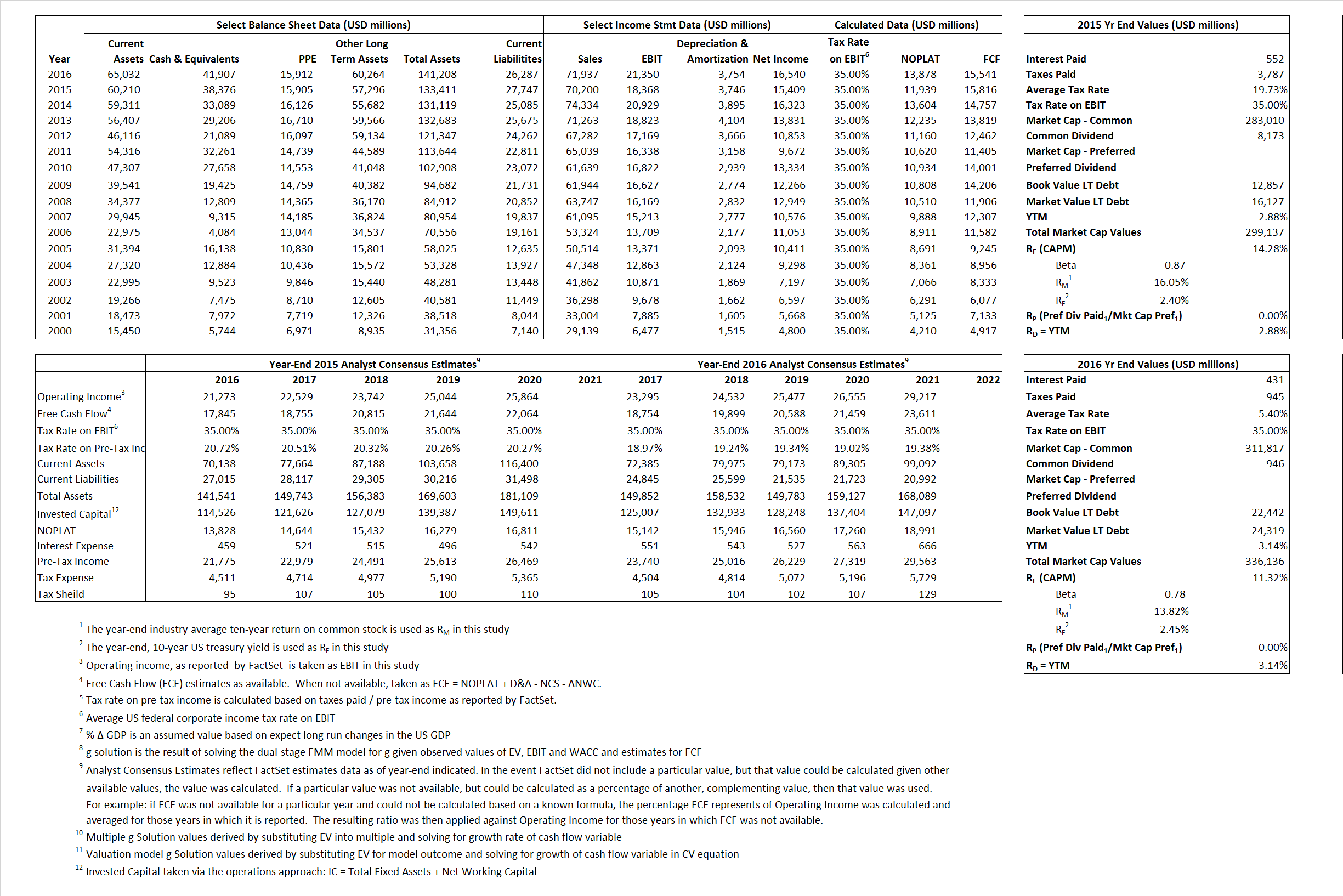# Johnson & Johnson

## Analyst Listing

The following analysts provide coverage for the subject firm as of May 2016:

 Broker Analyst Analyst Email Morningstar Damien Conover damien.conover@morningstar.com BTIG Dane Leone dleone@btig.com Leerink Partners Danielle Antalffy danielle.antalffy@leerink.com Argus Research David Toung dtoung@argusresearch.com Societe Generale Florent Cespedes florent.cespedes@sgcib.com RBC Capital Markets Glenn Novarro glenn.novarro@rbccm.com Raymond James Jayson Bedford jayson.bedford@raymondjames.com Jefferies Jeffrey Holford jholford@jefferies.com BMO Capital Markets Joanne K. Wuensch joanne.wuensch@bmo.com Cowen & Company Joshua Jennings josh.jennings@cowen.com Deutsche Bank Research Kristen Stewart kristen.stewart@db.com Wells Fargo Securities Larry Biegelsen lawrence.biegelsen@wellsfargo.com Piper Jaffray Richard J. Purkiss richard.j.purkiss@pjc.com Stifel Nicolaus Rick Wise danielle.antalffy@leerink.com Erste Group Stephan Lingnau stephan.lingnau@erstegroup.com Atlantic Equities Steve Chesney s.chesney@atlantic-equities.com Guggenheim Securities Tony Butler tony.butler@guggenheimpartners.com Credit Suisse Vamil Divan vamil.divan@credit-suisse.com

## Primary Input Data## Derived Input Data

### Equational Form

Net Operating Profit Less Adjusted Taxes NOPLAT  11,939 13,878$NOPLAT\, =\, EBIT\, x\, (1 \,-\, Avg \,\,Tax\,\, Rate\,\, on\,\, EBIT)$
Free Cash Flow FCF 15,816 15,541$FCF\,=NOPLAT\,+\,Non-Cash\,Expenses-\Delta NWC\,-\,NCS$
Tax Shield TS 109 23$TS\,=\,Interest\,\,Paid\,\,x\,\, Avg \,\,Tax\,\,Rate\,\, on\,\, Pre-Tax\,\, Income$
Invested Capital IC  105,664 114,921$IC\,=\,Fixed\,\,Operating\,\,Assets\,\,+\,\,Net\,\, Working\,\, Capital$
Return on Invested Capital ROIC 11.30% 12.08%$ROIC\,=\,\frac { NOPLAT }{ IC }$
Net Investment NetInv 3,376  13,011$NetInv\,=\,{ {IC}_{1}}-{{IC}_{0}}+Depreciation$
Investment Rate IR 28.28% 93.76%$IR\,=\,\frac {NetInv}{NOPLAT}$
Weighted Average Cost of Capital
WACCMarket  13.63% 10.71%$WACC\,=\,\frac { E }{ V } { R }_{ E }\,+\,\frac { P }{ V } { R }_{ P }\,+\,\frac { D }{ V } { R }_{ D }\left( 1- Avg\,\, Tax\,\,Rate\,\,on\,\,Pre-Tax\,\,Income \right)$
WACCBook   8.01%   7.10%
Enterprise value
EVMarket  260,761 294,229$EV\,=\,Market\,\,Cap\,\,Equity\,+\,\,Long\,\,Term\,\,Debt\,-\,Cash$
EVBook  253,960  292,352
Long-Run Growth
g = IR x ROIC
3.20%  11.32% Long-run growth rates of the income variable are used in the Continuing Value portion of the valuation models.
g = %$\Delta$ GDP   2.50%   2.50%
Margin from Operations M   26.17%  29.68%$M\,\,=\,\,\frac{EBIT}{SALES}$
Depreciation/Amortization Rate D   16.94%  14.95%$D\,\,=\,\,\frac{D+A}{EBITDA}$

## Valuation Multiple Outcomes

The outcomes presented in this study are the result of original input data, derived data, and synthesized inputs.

### model g solution

12/31/2015 12/31/2016 12/31/2015 12/31/2016 12/31/2015 12/31/2016

EV/SALES$\frac {EV}{Sales} \,= \,\frac{ROIC\, -\, g}{ROIC\,(WACC\,-\,g)}\,(1\,-\,T)\,(M)$

3.71  4.09  15.22%  9.84%  14.59%  10.30%

EV/EBITDA$\frac {EV}{EBITDA} \,= \,\frac{ROIC\, -\, g}{ROIC\,(WACC\,-\,g)}\,(1\,-\,T)\,(1\,-\,D)$

11.79 11.72 15.22%  9.84% 14.59% 10.30%

EV/NOPLAT$\frac {EV}{NOPLAT} \,= \,\frac{ROIC\, -\, g}{ROIC\,(WACC\,-\,g)}$

21.84 21.20 15.22% 9.84% 14.59% 10.30%

EV/FCFOPS$\frac {EV}{FCF_{OPS}} \,= \,\frac{ROIC\, -\, g}{ROIC\,(WACC\,-\,g)}\,(1\,-\,T)$

16.49 18.93 15.22% 9.84% 14.59% 10.30%

EV/EBIT$\frac {EV}{EBIT} \,= \,\frac{ROIC\, -\, g}{ROIC\,(WACC\,-\,g)}\,(1\,-\,T)$

14.20 13.78 15.22% 9.84% 14.59% 10.30%

EV/IC$\frac {EV}{IC} \,= \,\frac{ROIC\, -\, g}{WACC\,-\,g}$

2.47 2.56 15.22% 9.84% 14.59% 10.30%